Programming

# Dynamically importing functions C++

This post will introduce you to a relatively simple concept, the purpose of this is to hide functions from the IAT and also call functions from Windows Native API.

The way this is done is by:

• Defining the prototype of the function (use MSDN for that)
• Loading the DLL (unless it is kernel32.dll) -> LoadLibrary
• Getting the handle of the module -> GetModuleHandle
• Getting the function address -> GetProcAddress

Stop here if you want to figure out how to actually code it

So let’s hop into coding!

The first example I’ll give is for CreateProcessW (check this if you don’t know what W means)

#### Defining the function’s prototype

By checking the MSDN documentation we come across this prototype:That green underline is due to VS not finding the definition of that function yet.

WINAPI is an alias for stdcall (a calling convention).

So, let’s arrange that, make it a function pointer and typedef: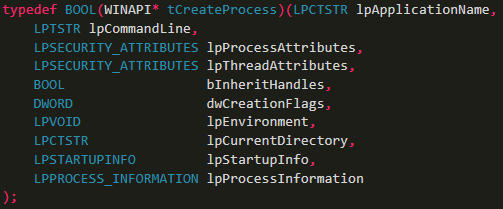Ps: Note that Asterisk after WINAPI, meaning it refers to a function pointer.

Now we write a variable of that type:Remember that writing the function pointer is optional but makes the code way cleaner.

The next step is getting the handle of Kernel32.dll since that’s where CreateProcessW lies (you can find that out by the library requirement on the MSDN page), we don’t need to load it since it gets automatically loaded into every process: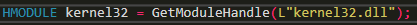#### Getting the function address

The final step before actually calling the function is to get the address of the function:#### Calling the function

Now let’s call it!#### Function from user32.dll

Now let’s try with MessageBoxW: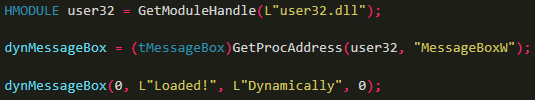Now, let’s run and…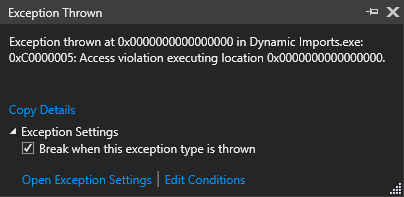Remember I said that we needed to load the DLL if we weren’t using a Kernel32 function? Yep, that’s right now instead of GetModuleHandle, we use LoadLibrary. (which also returns the module’s handle)And now it works!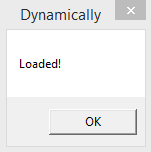Now I believe you can try to call this native function “NtGetTickCount”: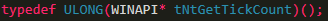The Nt implies it is from ntdll.dll being a native function

And that’s it! See? Not rocket science 😛